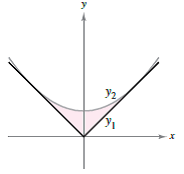Chapter 7.1, Problem 80E

Chapter
Section
Textbook Problem

Mechanical Design The surface of a machine part is the region between the graphs of y 1 = | x |   and   y 2 = 0.08 x 2 + k (see figure).(a) Find it such that the parabola is tangent to the graph of y 1 .(b) Find the area of the surface of the machine part.

a)

To determine

To Calculate: The value of ‘k’ if the parabola is tangent to the graph of the curve y1.

Explanation

Given:

The surface of a machine part is the region bounded between the graphs of the curves y1=|x| and y2=0.08x2+k

As shown in figure below:

Formula Used:

Slope of the curve y2 at the point (x0,y0) is 0.16x0

Since functions are even,

Thus the region bounded by curves is symmetric about Y-axis.

So, we consider only first quadrant y1=x.....(1)

If the point (x0,y0) is the point of tangency then from equation (1) y0=x0.

Slope of the tangent line at (x0,y0) is one because it is same at any point of the curve.

Calculation:

Consider the provided figure as given below:

y1=|x| and y2=0.08x2+k

Since the functions are even,

Thus the region bounded by these curves is symmetric about Y-axis.

So, consider only 1st quadrant.

Thus, y1=x ….....(1) x0

b)

To determine

To Calculate: The area of the surface of the machine part.

Still sussing out bartleby?

Check out a sample textbook solution.

See a sample solution

The Solution to Your Study Problems

Bartleby provides explanations to thousands of textbook problems written by our experts, many with advanced degrees!

Get Started

Convert the expressions in Exercises 6584 to power form. x2y

Finite Mathematics and Applied Calculus (MindTap Course List)

In problems 5-8, Use a calculator to evaluate the indicated powers. 5.

Mathematical Applications for the Management, Life, and Social Sciences

If f(x) = 2x + In x, find f1(2).

Single Variable Calculus: Early Transcendentals